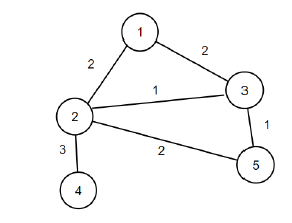# C++ Program to find out the maximum amount of score that can be decreased from a graph

Suppose, there is a weighted, undirected graph that has n vertices and m edges. The score of the graph is defined as the addition of all the edges weights in the graph. The edge weights can be negative, and if they are removed the score of the graph increases. What we have to do, we have to make the score of the graph minimum by removing the edges from the graph while keeping the graph connected. We have to find out the maximum amount of score that can be decreased.

The graph is given in an array 'edges', where each element is of the form {weight, {vertex1, vertex2}}.

So, if the input is like n = 5, m = 6, edges = {{2, {1, 2}}, {2, {1, 3}}, {1, {2, 3}}, {3, {2, 4}}, {2, {2, 5}}, {1, {3, 5}}}, then the output will be 4.If we remove edge (1, 2) and (2, 5) from the graph, the total decrease in score will be 4 and the graph will stay connected.

To solve this, we will follow these steps−

cnum := 0
Define an array par of size: 100.
Define an array dim of size: 100.
Define a function make(), this will take v,
par[v] := v
dim[v] := 1
Define a function find(), this will take v,
if par[v] is same as v, then:
return v
return par[v] = find(par[v])
Define a function unify(), this will take a, b,
a := find(a)
b := find(b)
if a is not equal to b, then:
(decrease cnum by 1)
if dim[a] > dim[b], then:
swap values of (a, b)
par[a] := b
dim[b] := dim[b] + dim[a]
cnum := n
sort the array edges based on edge weights
for initialize i := 1, when i <= n, update (increase i by 1), do:
make(i)
res := 0
for each edge in edges, do:
a := first vertex of edge
b := second vertex of edge
weight := weight of edge
if find(a) is same as find(b), then:
if weight >= 0, then:
res := res + 1 * weight
if cnum is same as 1, then:
if weight >= 0, then:
res := res + 1 * weight
Otherwise
unify(a, b)
return res

## Example

Let us see the following implementation to get better understanding −

#include <bits/stdc++.h>
using namespace std;

int cnum = 0;
int par;
int dim;

void make(int v){
par[v] = v;
dim[v] = 1;
}
int find(int v){
if(par[v] == v)
return v;
return par[v] = find(par[v]);
}
void unify(int a, int b){
a = find(a); b = find(b);
if(a != b){
cnum--; if(dim[a] > dim[b]){
swap(a, b);
}
par[a] = b; dim[b] += dim[a];
}
}
int solve(int n, int m, vector <pair <int, pair<int,int>>> edges){
cnum = n;
sort(edges.begin(), edges.end());
for(int i = 1; i <= n; i++)
make(i);
int res = 0;
for(auto &edge : edges){
int a = edge.second.first;
int b = edge.second.second;
int weight = edge.first;
if(find(a) == find(b)) {
if(weight >= 0)
res += 1 * weight;
continue;
}
if(cnum == 1){
if(weight >= 0)
res += 1 * weight;
} else{
unify(a, b);
}
}
return res;
}
int main() {
int n = 5, m = 6;
vector <pair<int, pair<int,int>>> edges = {{2, {1, 2}}, {2, {1, 3}}, {1, {2, 3}}, {3, {2, 4}}, {2, {2, 5}}, {1, {3, 5}}};
cout<< solve(n, m, edges);
return 0;
}

## Input

5, 6, {{2, {1, 2}}, {2, {1, 3}}, {1, {2, 3}}, {3, {2, 4}}, {2, {2, 5}}, {1, {3, 5}}}


## Output

4